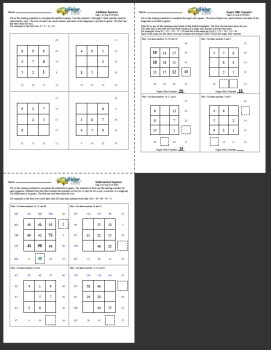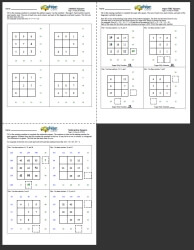Worksheets and No Prep Teaching Resources Math Make Puzzles, Critical ThinkingMixed Math Square Puzzles Printable Activity Lesson Plan

Mixed Math Square Puzzles BookAddition Squares, Super Silly Number Squares, and Subtraction Squares (3 pages per key; 1 key) Addition Squares, Super Silly Number Squares, and Subtraction Squares (3 pages per key; 3 keys)

Super Silly Squares

Subtraction Squares

Multiplication Squares

Magic Square Puzzles

Have a suggestion or would like to leave feedback?